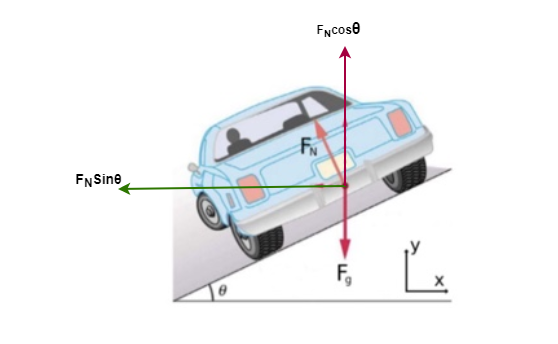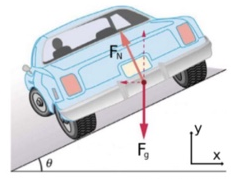# Problem: Circular turns of radius r in a race track are often banked at an angle to allow the cars to achieve higher speeds around the turns. Assume friction is not present, and use the coordinate system specified.Find y component of the normal force FN of a car going around the turn in terms of the angle θ and the magnitude of the normal vector FN?

###### FREE Expert Solution

Consider the free-body diagram below:From the free-body diagram, the y component of the normal force, FN is:

FN,y = FNcosθ

99% (135 ratings)###### Problem Details

Circular turns of radius r in a race track are often banked at an angle to allow the cars to achieve higher speeds around the turns. Assume friction is not present, and use the coordinate system specified.Find y component of the normal force FN of a car going around the turn in terms of the angle θ and the magnitude of the normal vector FN?